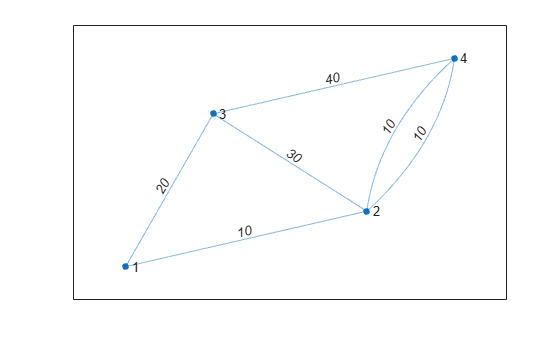# findedge

Locate edge in graph

## Syntax

``[sOut,tOut] = findedge(G)``
``[sOut,tOut] = findedge(G,idx)``
``idxOut = findedge(G,s,t)``
``[idxOut,m] = findedge(G,s,t)``

## Description

example

````[sOut,tOut] = findedge(G)` returns the source and target node IDs, `sOut` and `tOut`, for all of the edges in graph `G`.```

example

````[sOut,tOut] = findedge(G,idx)` finds the source and target nodes of the edges specified by `idx`.```

example

````idxOut = findedge(G,s,t)` returns the numeric edge indices, `idxOut`, for the edges specified by the source and target node pairs `s` and `t`. The edge indices correspond to the rows `G.Edges.Edge(idxOut,:)` in the `G.Edges` table of the graph. If there are multiple edges between `s` and `t`, then all their indices are returned. An edge index of `0` indicates an edge that is not in the graph.```
````[idxOut,m] = findedge(G,s,t)` additionally returns a vector `m` indicating which node pair `(s,t)` is associated with each edge index in `idxOut`. This is useful when there are multiple edges between the same two nodes.```

## Examples

collapse all

Create a graph, and then determine the edge index for the (1,2) and (3,5) edges.

```s = [1 1 2 2 2 3 3 3]; t = [2 3 3 4 5 6 7 5]; G = graph(s,t)```
```G = graph with properties: Edges: [8x1 table] Nodes: [7x0 table] ```
`idxOut = findedge(G,[1 3],[2 5])`
```idxOut = 2×1 1 6 ```

`idxOut` contains the row index into `G.Edges.EndNodes` for each specified edge.

Create a graph, and then determine the end nodes of all edges in the graph.

```s = {'a' 'a' 'b' 'b' 'c' 'c'}; t = {'b' 'c' 'd' 'e' 'f' 'g'}; G = graph(s,t); G.Edges```
```ans=6×1 table EndNodes ______________ {'a'} {'b'} {'a'} {'c'} {'b'} {'d'} {'b'} {'e'} {'c'} {'f'} {'c'} {'g'} ```
`[sOut,tOut] = findedge(G)`
```sOut = 6×1 1 1 2 2 3 3 ```
```tOut = 6×1 2 3 4 5 6 7 ```

Create a graph, and then determine the end nodes for the edges whose indices are `3` and `7`.

```s = [1 1 1 1 2 2 3 3 4 4]; t = [2 3 4 5 6 7 8 9 10 11]; G = digraph(s,t)```
```G = digraph with properties: Edges: [10x1 table] Nodes: [11x0 table] ```
`[sOut,tOut] = findedge(G,[3 7])`
```sOut = 2×1 1 3 ```
```tOut = 2×1 4 8 ```

Create a graph.

```s = [1 1 2 3]; t = [2 3 3 4]; weights = [10 20 30 40]; G = graph(s,t,weights)```
```G = graph with properties: Edges: [4x2 table] Nodes: [4x0 table] ```

Find the weight of the (1,3) edge, using `findedge` to retrieve the index.

`G.Edges.Weight(findedge(G,1,3))`
```ans = 20 ```

Use `findedge` to change the weights of several multigraph edges.

Create and plot a multigraph. This graph has two edges between node 2 and node 4.

```s = [1 1 2 3 2 2]; t = [2 3 3 4 4 4]; weights = [10 20 30 40 10 10]; G = graph(s,t,weights); plot(G,'EdgeLabel',G.Edges.Weight)```Change the weights of the edges between nodes (3,2) and (2,4). Specify two outputs to `findedge` to get the end-node indices, `m`. This output is useful when there are multiple edges between two nodes, since `idxOut` can have more elements than the number of node pairs in `s` and `t`. The edge `idxOut(1) = 3` connects the node pair `(s(1),t(1)) = (3,2)`, and the edges `idxOut(2) = 4` and `idxOut(3) = 5` connect the edge `(s(2),t(2)) = (2,4)`.

```s = [3 2]; t = [2 4]; w = [1 4]; [idxOut, m] = findedge(G, s, t)```
```idxOut = 3×1 3 4 5 ```
```m = 3×1 1 2 2 ```
```G.Edges.Weight(idxOut) = w(m); plot(G,'EdgeLabel',G.Edges.Weight)```## Input Arguments

collapse all

Input graph, specified as either a `graph` or `digraph` object. Use `graph` to create an undirected graph or `digraph` to create a directed graph.

Example: `G = graph(1,2)`

Example: `G = digraph([1 2],[2 3])`

Node pairs, specified as separate arguments of node indices or node names. Similarly located elements in `s` and `t` specify the source and target nodes for edges in the graph.

This table shows the different ways to refer to one or more nodes either by their numeric node indices or by their node names.

FormSingle NodeMultiple Nodes
Node index

Scalar

Example: `1`

Vector

Example: `[1 2 3]`

Node name

Character vector

Example: `'A'`

Cell array of character vectors

Example: `{'A' 'B' 'C'}`

String scalar

Example: `"A"`

String array

Example: `["A" "B" "C"]`

Categorical array

Example: `categorical("A")`

Categorical array

Example: `categorical(["A" "B" "C"])`

Example: `G = findedge(G,[1 2],[3 4])`

Example: ```G = findedge(G,{'a' 'a'; 'b' 'c'},{'b' 'c'; 'c' 'e'})```

Edge indices, specified as a scalar or vector of positive integers. The edge index corresponds to a row in the `G.Edges` table of the graph, `G.Edges(idx,:)`.

## Output Arguments

collapse all

Edge indices, returned as a scalar or vector of nonnegative integers. The edge indices correspond to rows in the `G.Edges` table of the graph, `G.Edges(idxOut,:)`. An edge index of `0` indicates an edge that is not in the graph.

The length of `idxOut` corresponds to the number of node pairs in the input, unless the input graph is a multigraph.

End node indices, returned as a vector. The values in `m` connect the edge indices in `idxOut` to the input node pairs `(s,t)`. The edge `idxOut(j)` connects the node pair with index `m(j)`.

Node IDs, returned as separate scalars or vectors of positive integers. Similarly located elements in `sOut` and `tOut` specify the source and target nodes that form the edges `G.Edges(idx,:)`.